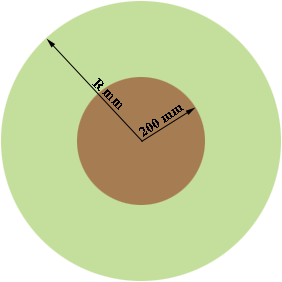SEARCH HOMEMath Central Quandaries & QueriesQuestion from truong: hi. i have trouble to calculate the diameter of the plastic roll. the sheet is 765 m long and 0.8 mm to wrap around the core 400 mm in dia. please help me with the formula to calculate the dia of plastic roll, thanks in advanceTruong,

I'm going to work in millimeters so you sheet is 765000 mm long and 0.8 mm thick. The edge of this sheet then forms a very long, thin rectangle with an area of 765000 × 0.8 = 612000 square mm. When wrapped around the core this rectangle then takes the shape of an annulus, the region between two concentric circles.The inside radius (the radius of the core) is 200 mm and suppose the outside radius is R mm. The area of this annulus is the difference in the areas of the two circles, so the area is

π R2 - π 2002 = π (R2 - 2002) square millimeters.

The area of the edge of the plastic sheet is the same whether it is seen as a rectangle or an annulus so

π (R2 - 2002) = 612000 square mm.

Thus

R2 = (612000/π) - 2002 = 234805.65 square mm

and hence

R = √234805.65 = 484.6 mm.

Thus the diameter of the roll is 2 × 484.6 = 969 mm

HarleyMath Central is supported by the University of Regina and The Pacific Institute for the Mathematical Sciences.